# Quiz 10: California Products

Estimate of change in "PFMOH" cost if one extra batch of 150,000 milk crates was to run: • Usually, the plant fixed manufacturing overhead (PFMOH) is a fixed expenditure. • This implies that it is based on the capacity, which is not used; therefore, running an extra batch of 150,000 milk crates will not bring any change in the plant fixed manufacturing overhead. • According to Exhibit 3 the plant fixed manufacturing overhead cost varies every month on the basis of the production of a large product mix. • The information regarding insurance, rent, taxes, and supply of the machines is not given; as such, the changes in the above-mentioned figures cannot be calculated. • The additional indirect cost is calculated; extract the information given in exhibit 3 and text: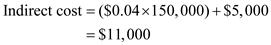Where, The total cost per crate is \$0.04 and the cost of the stamping machine acquired is \$5000. Thus the additional indirect cost for the labor and machine to stamp the crates is \$11,000 • The additional cost for loading the crates for shipping is calculated by extracting the information given in the text as follows: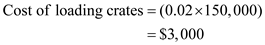Where, The labor cost per crate is \$0.02. Thus the additional cost for loading the crates for shipping is \$3,000.
Estimate of the incremental cost per unit for one batch of 150,000 milk crates: Incremental cost: This is defined as the decrease or increase in the value of the costs as an outcome of one less or one more unit of output produced. By extracting the information: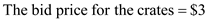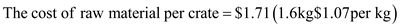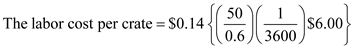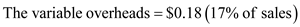Calculation of incremental cost per unit: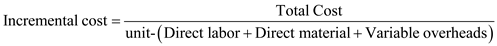Where, The total cost per unit is \$3, the direct labor cost per crate is \$0.14, the direct material cost per crate is \$1.71, and the variable overhead is \$0.18.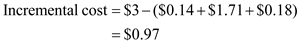Thus, the additional incremental cost is \$0.97.
By extracting information: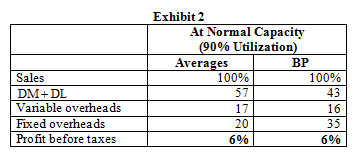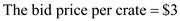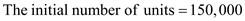Calculate the normal price for milk crates for an average job shop: Step-1: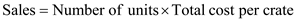Substitute: The number of units is 150,000 The total cost per crate is \$3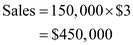Thus, the amount of sales is \$450,000. Step-2: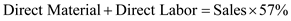Substitute: The amount of sales is \$450,000: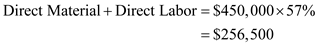Thus, the amount of direct material and labor is \$256,500. Step-3: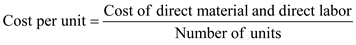Substitute: The cost of direct material and labor is \$256,500 and the number of units is 150,000:Thus, the normal price for milk crates for an average job shop is \$1.71. Suggestion regarding the initial bid price of \$3: From the above calculation, we can conclude that the price for an average job shop is \$1.71 and the Brunswick Plastics is given as \$3. This implies that there is a fair chance of losing the bid on the contract, as \$3 is significantly higher than the average price of \$1.71.